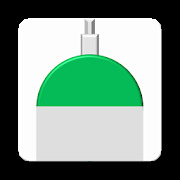#### Alphabetical

A | B | C | D | E | F | G | H | I | J | K | L | M | N | O | P | Q | R | S | T | U | V | W | X | Y | Z

# Oxygen Duration CalculatorCalculate the number of minutes remaining in an oxygen tank based on tank size, pressure and continuous flow rate.The following equation is used to determine the remaining duration of flow:<br><br>Duration of Flow = Oxygen Tank Conversion Factor * (Remaining Tank Pressure - Safe Residual Pressure) / Continuous Flow Rate<br><br>Oxygen Cylinder Conversion Factors<br>• D Tank = 0.16<br>• E Tank = 0.28<br>• G Tank = 2.41<br>• H/K Tank = 3.14<br>• M tank = 1.56<br><br>Safe Residual Pressure = 200psi